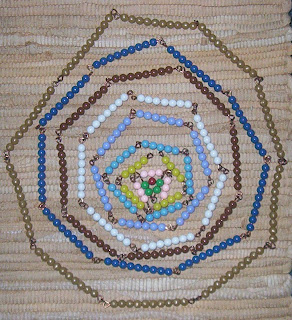Geometry is a very hands-on experience in Montessori. Nothing theoretical and everything practical here!

Primary:
There is no explicit geometry table of contents for primary. Geometric concepts are explored through the use of the following inexhaustible list of experiences:

Mathematics:
Golden beads (point, line, plane, 3D that becomes a point again)
Wooden Hierarchical Material

Language:
Environment Language Games
Card materials
Cutting and other art experiences
Metal Insets (design work - preparation for writing)

Exercises of Practical Life:
visual layout of materials
design work on materials and in art pieces
variety of shapes available in the environment

Sensorial:Geometry Cabinet
Geometry Cards
Leaf Cabinet
Leaf Cards
Constructive Triangles
Rectangular Box A
Rectangular Box B
Triangular Box
Large Hexagonal Box
Small Hexagonal Box
12 Blue Triangles
Binomial Cube
Trinomial Cube
Decanomial Square
Knobless Cylinders
Geometric Solids
Metal Insets (design work)

I. Introduction to Geometry
Original Geometry Story
II. Congruency, Similarity, Equivalency I
Iron Material
Constructive Triangles/Blue Box
III. Polygons
Polygons
IV. Angles I
Angles
Parts of an Angle
How to Measure an Angle
Regular Protractor (including bisecting an angle)
V. Lines
Nomenclature
Position of Two Lines
Intersecting Lines
VI. Angles II
Relationships Between Angles
Rel Bet Angles Formed by Parallel Lines Cut by a Transversal
Size Relationships of These Angles
VII. Equivalency II
Pythagoras Plates I and II
Pythagoras with the Constructive Triangles
VIII. Polygons II
Nomenclature of Polygons
Sum of the Angles in Plane Figures
IX. Equivalency III
Equivalency with Iron Material
Euclid’s Plate
X. Area
Concept of Area
Deriving Formulae with the Yellow Material
Deriving Formulae with the Iron Material
XI. Circle I
The Circle Nomenclature
Relationship Between Lines and Circumferences
Relationships Between Two Circumferences
XII. Circle II
Area of a Circle:
Circle as a Special Polygon
Measuring the Circumference
Formula for Area of a Circle
Relationship Between the Apothem and Side of a Plane Figure
XIII. Solid Geometry
Concept of Volume
Equivalence as Related to Solid Figures
Three Important Dimensions
Equivalence Between Prisms with Various Bases
Derivation of the Formula
Solids of Rotation
Volume of the Pyramid
Volume of the Cylinder and Cone
Polyhedrons
Lateral and Total Surface Area of Solids
Geometry Nomenclature Material
Geometry Commands
Geometry Summary
XV. Appendix
Geometry Charts
Geometry Stories